# S16E3 - Spontaneous Reactions and the Gibbs Free Energy Equation

## Gibbs Free Energy, G

Gibbs Free Energy = a thermodynamic function that not only is related to spontaneity, but also deals with the temperature dependence of spontaneity:

### ΔG  =  ΔH  -  TΔS

Note: all functions are from the point of view of the "system" (ΔGsys, ΔHsys, ΔSsys)

➞  if ΔG < 0, the process is spontaneous (at constant T and P)

➞  if ΔG > 0, the process is not spontaneous (at constant T and P)

----------

To calculate ΔG, you must compare and consider the ΔH and ΔS values, and you must consider the kelvin temperature (T).

ex:  At what temperatures is the following process spontaneous at 1 atm?

Br2(l)  →  Br2(g)

ΔH° = 31.0 kJ/mol
ΔS° = 93.0 J/K.mol

Also, what's the normal boiling point of Br2(l)?
_________

➞  ΔG must be negative (-) for the process to be spontaneous

➞  We can find the threshold temperature (T) by setting ΔG° = 0...So, the threshold temperature is 333 K.

Above 333 K, the forward reaction is spontaneous because at higher temperatures, the "TΔS" term is dominant in:

ΔG°  =  ΔH°  -  TΔS°

When T = 333 K, the free energy change (ΔG) = 0 and the liquid and gas phases of Br2 coexist at 1 atm.

Therefore, the normal boiling point (bp) is also 333 K.

==========

## Entropy Changes (ΔS) in Chemical Reactions

TIP - Focus on the amount of gas molecules or atoms.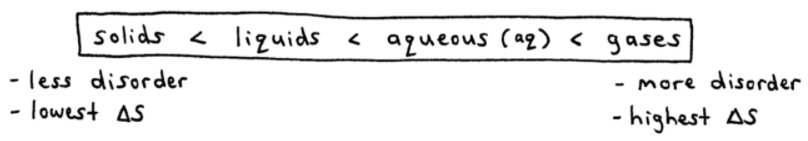Gases - highest level of disorder, so highest amount of entropy (ΔS)

Solids - lowest level of disorder, so lowest amount of entropy (ΔS)

----------

ex:  Does entropy increase or decrease in the following reactions?

a.     N2(g)  +  3H2(g)  →  2NH3(g)

➞  4 gas molecules "goes to" 2 gas molecules, and fewer gas molecules means fewer possible configurations.

➞  fewer gas molecules  =  less disorder, so entropy decreases,   ΔS < 0

b.     4NH3(g)  +  5O2(g)  →  4NO(g)  +  6H2O(g)

➞  9 gas molecules "goes to" 10 gas molecules, and more gas molecules means more possible configurations.

➞  more gas molecules  =  more disorder, so entropy increases,   ΔS > 0

==========

## Standard Entropy Values (S°)

Standard Entropy Values (S°) are listed in a lengthy table or appendix in the back of your textbook.

Or you can find them here:  List of Entropy Values

- Notice that indeed S° increases for a substance in going from s  g

- S° has units of " J/K.mol "

- To calculate ΔS° for a reaction:I've boxed up the 2nd version of the entropy equation above for a reason.  It's easier to remember.

So use that one!

----------

ex:  Calculate ΔS° for this reaction:

Al2O3(s)  +  3H2(g)  →  2Al(s)  +  3H2O(g)
_________

➞  ΔS°rxn  =  [sum of product entropy values]  -  [sum of reactant entropy values]

➞  don't forget to take # of moles of each substance into account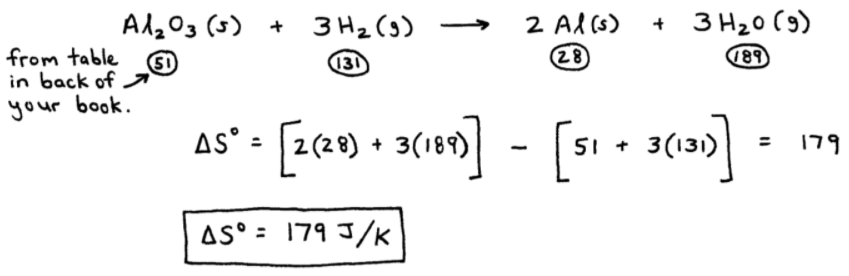----------

QUESTION - In the above reaction, 3 gas molecules "goes to" 3 gas molecules.

So how come the ΔS° value is large (179 J/K) instead of nearly 0 J/K ?...

➞  ANSWER - the large ΔS° value is because H2O is a more complex molecule than H2, and thus has a higher standard entropy value (S°).

Why?  Because H2O's molecular structure, being more sophisticated, has a greater number of vibrations/rotations/etc. which increases its randomness and disorder (entropy).

==========

## Free Energy (G) and Chemical Reactions

The standard free energy change (ΔG°) is the change in free energy that occurs when the reactants in their standard states are converted to products in their standard states.

Knowing ΔG° is useful because the more negative its value is, the more likely it is that a reaction will occur (or "shift right" to reach equilibrium).

----------

### There are 3 Ways to Calculate ΔG°

1.  Using equation:  ΔG°  =  ΔH°  -  TΔS°  (at constant T, P)

➞ where both ΔH° and ΔS° are calculated using a Table of Thermodynamic Values.

2.  Using procedures similar to Hess' Law (see "Section 6")

ex:  From the data provided below, calculate ΔG° for this reaction:

2 CO(g)  +  O2(g)  →  2 CO2(g)

Data: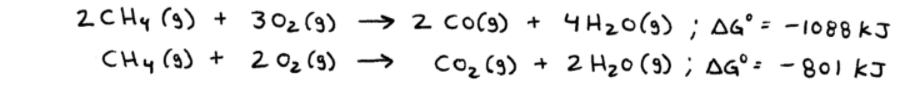_________

➞  the only way to get " 2 CO(g) " as a reactant is to flip the first reaction.  So...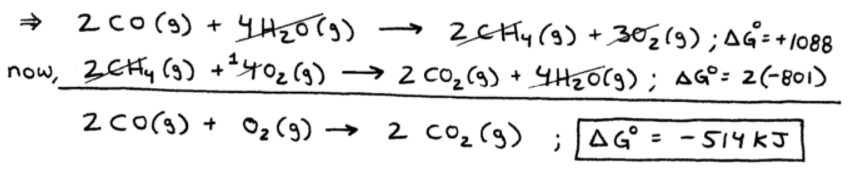3.  Using standard free energies of formation (ΔGf°)

ΔGf°  =  change in free energy that accompanies the formation of 1 mole of substance from its elements, with all of the reactants and products in their standard states.

You can find them here:  List of Free Energy Values, Gf°

➞  value of ΔGf° = 0 for elements in their standard states.

➞  To calculate ΔG° for a reaction: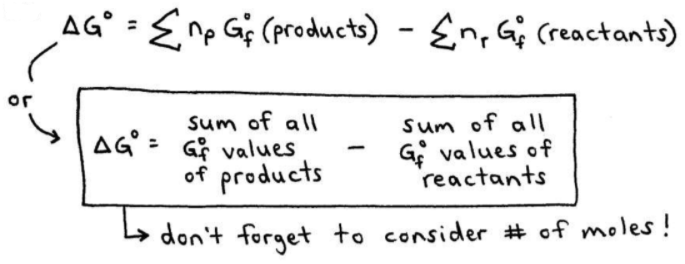Look familiar?  Use the boxed up version.

It's easier to remember...

----------

ex:  Is the following reaction spontaneous under standard conditions?

C2H4(g)  +  H2O(l)  →  C2H5OH(l)
_________